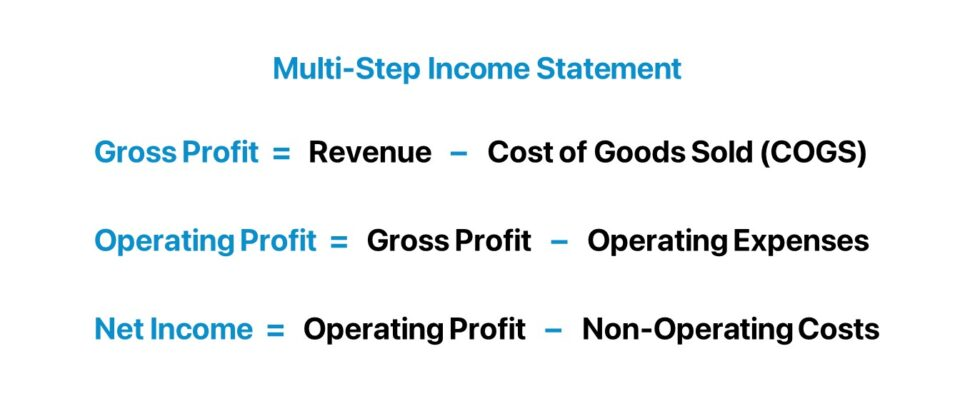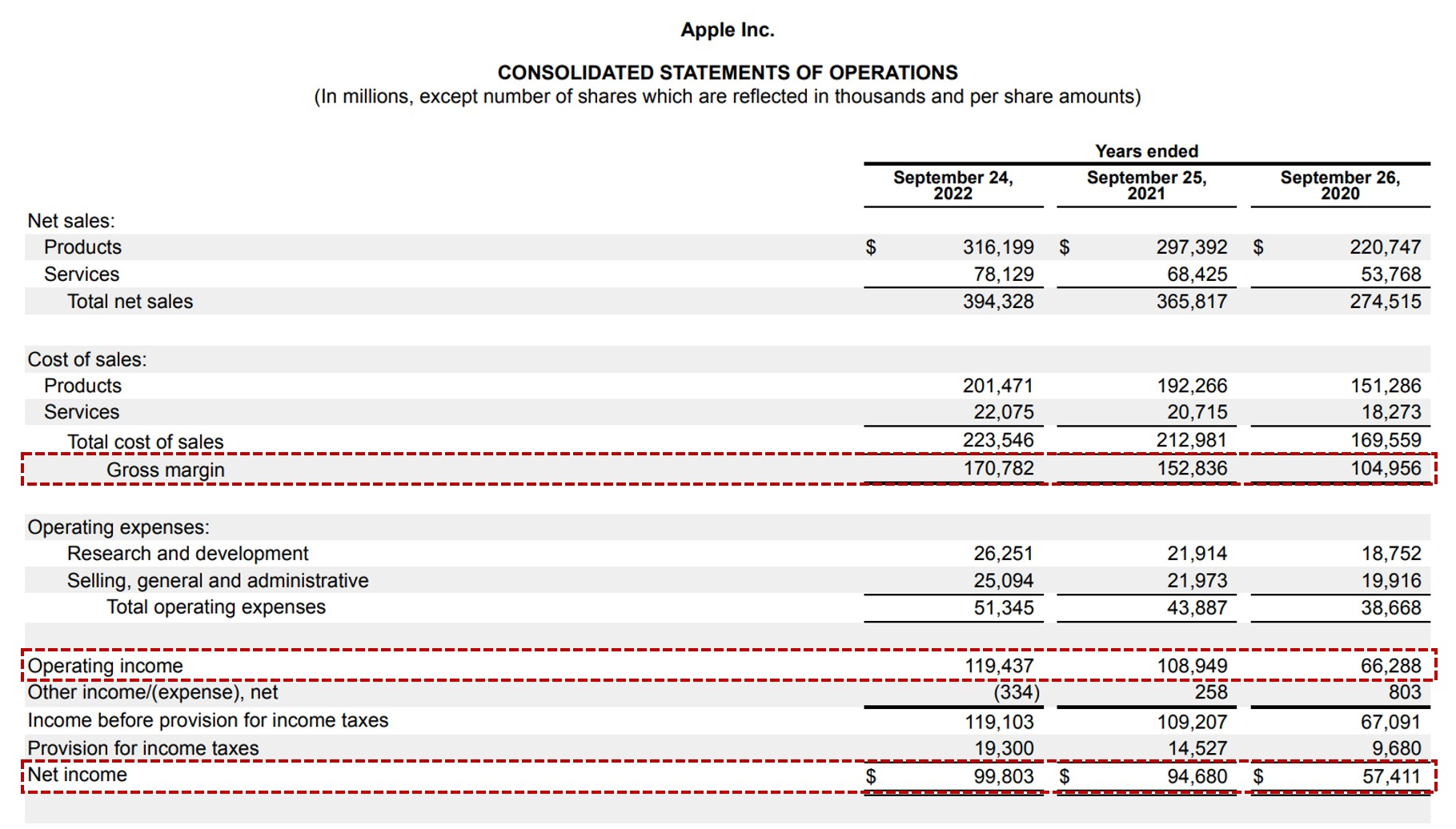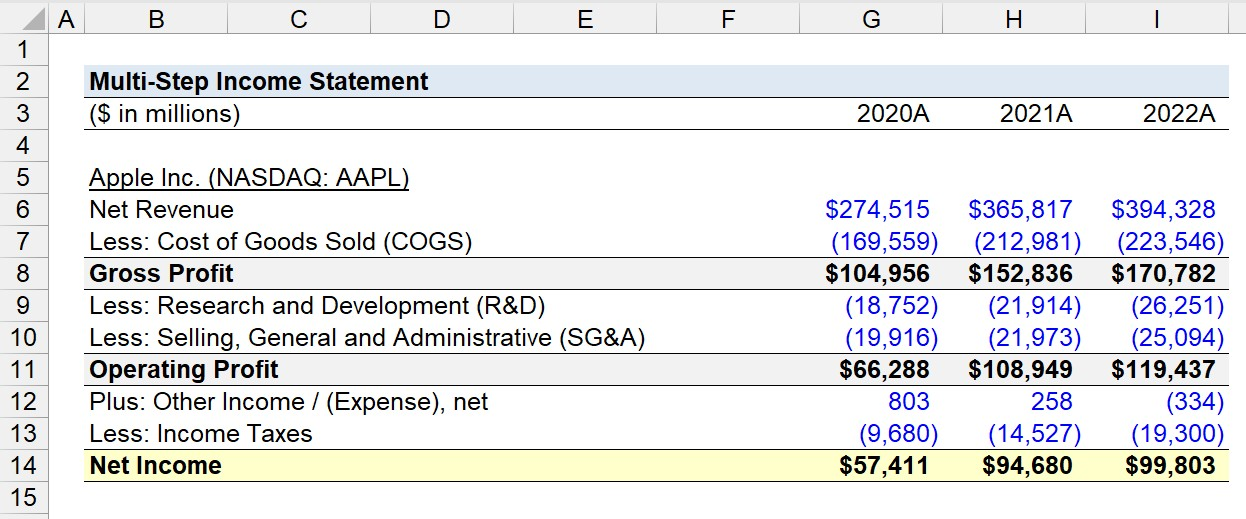Welcome to Wall Street Prep! Use code at checkout for 15% off.# Multi-Step Income Statement

Guide to Understanding the Multi-Step Income Statement## What are the Components of a Multi-Step Income Statement?

The multi-step income statement is a method of presenting the financial results of a company by segmenting the revenue, costs, and expenses into distinct sections.

The components of the multi-step income statement comprise three equations that calculate a profit metric that each measures a unique attribute of the underlying company’s financial performance.

By separating the income statement, or profit and loss statement (P&L), into multiple sections, the presentation of the financial statement offers more in-depth information on the company’s performance to facilitate a more detailed analysis to better comprehend its business model and operating drivers.

In particular, there are three equations that underpin the multi-step income statement:

1. Gross Profit → The gross profit is a profit metric that reflects the earnings remaining after a company’s cost of goods sold (COGS) is deducted from its net revenue.
2. Operating Profit (EBIT) → The operating profit, or “EBIT,” is a profitability metric that measures the income remaining once operating expenses have been subtracted from a company’s gross profit.
3. Net Income → The net income, or after-tax earnings, is an accounting measure of profitability where all costs, operating and non-operating costs, have been deducted from net revenue.

## How to Prepare a Multi-Step Income Statement?

### Step 1. Gross Profit Equation

Starting off, the gross profit is equal to the revenue generated by a company in a pre-defined period minus its cost of goods sold (COGS), which are the direct costs incurred as part of its core business operations.

Gross Profit = Revenue Cost of Goods Sold (COGS)

The COGS line item includes the direct costs of producing the goods or services sold by a company to produce revenue, such as the following:

• Cost of Raw Materials
• Cost of Direct Labor
• Purchase of Inventory or Merchandise

### Step 2. Operating Profit Equation

From the gross profit line item, the next profit measure is the operating profit, or “EBIT,” an abbreviation for “Earnings Before Interest and Taxes.”

Operating Profit (EBIT) = Gross Profit Operating Expenses

Like COGS, operating expenses are an integral part of the core operating activities of a company. However, operating expenses are not directly related to the revenue model of the company.

Some of the more common examples of operating expenses are as follows.

• Selling, General and Administrative (SG&A)
• Research and Development (R&D)
• Sales and Marketing (S&M)

### Step 3. Net Income Equation

The third and final component of the multi-step income statement is net income (the “bottom line”), which represents the net profitability of a company per accrual accounting standards.

The net income metric is inclusive of all costs – operating and non-operating costs – in contrast to the operating profit metric, which only accounts for operating costs (i.e. COGS and Opex).

Therefore, the net income factors in non-operating items such as interest expense, other non-operating costs such as non-recurring losses from inventory write-downs, and income taxes paid to the government.

The net income equation is as follows.

Net Income = Operating Profit Non-Operating Costs

Contrary to operating costs, non-operating costs are not part of the core, recurring operating activities of a company.

For instance, interest expense is a non-operating cost since the item pertains to the financing activities of a company rather than any of its specific operating activities.

## Single-Step vs. Multiple-Step Income Statement: What is the Difference?

The difference between the single-step and multiple-step income statement is as follows.

• Single-Step Income Statement → A single-step income statement is the simplest, easiest-to-understand format to present the financial performance of a company, which is most often used for small businesses. Under the single-step income statement format, a single equation is used to arrive at a company’s net income for the given period.
• Multiple-Step Income Statement → The multi-step income statement, on the other hand, abides by a three-step process to compute net income, where each section serves a unique purpose.

Fundamentally, the basic premise of either presentation format is conceptually the same, granted the outcome of either method is to arrive at net income.

Yet, from a practical perspective, the insights that can be obtained from the multi-step income statement are ultimately far more insightful to understand and analyze the financial performance of a company.

Under the single-step income statement, the core equation is as follows.

Net Income = Revenue (Costs + Expenses)

While the single-step format might be sufficient for certain small companies, such as a local family-owned business, the decision to raise capital from investors or to borrow debt capital from lenders causes the reporting style to become impractical.

In short, the introduction of stakeholders for the first time is typically the catalyst for private companies to transition from the single-step to multiple-step income statement. For public companies, there are strict reporting guidelines established where a single-step format is not even an option.Apple Income Statement (Source: AAPL FY-2022 10-K)

## Multi-Step Income Statement Calculator

We’ll now move to a modeling exercise, which you can access by filling out the form below.#### Excel TemplateSubmitting...

## Multi-Step Income Statement Calculation Example

Suppose we’re tasked with preparing a multi-step income statement for Apple (NASDAQ: AAPL) for fiscal years ending 2020 to 2022.

Based on the latest 10-K filing of Apple (AAPL), we’ll input the historical income statement data into our spreadsheet using the proper formatting convention, in which hard-coded figures are entered in blue font while calculations are left in black font.

The first step is to compute the gross profit of Apple in each period using the following set of equations:

• Gross Profit – 2020A = \$274,515 million + (169,559 million) = \$104,956 million
• Gross Profit – 2021A = \$365,817 million + (212,981 million) = \$152,836 million
• Gross Profit – 2022A = \$394,328 million + (223,546 million) = \$170,782 million

Given the gross profit of Apple for each period, the next step is to subtract operating expenses to determine the company’s operating profit in each fiscal year.

• Operating Profit – 2020A = \$104,956 million + (18,752 million) + (19,916 million) = \$66,288 million
• Operating Profit – 2021A = \$152,836 million + (21,914 million) + (21,973 million) = \$108,949 million
• Operating Profit – 2022A = \$170,782 million + (26,251 million) + (25,094 million) = \$119,437 million

In the final step, the remaining equation solves for the net income of Apple in our trailing three-year period.

• Net Income – 2020A = \$66,288 million + \$803k + (\$9,680 million) = \$57,411 million
• Net Income – 2021A = \$108,949 million + \$258k + (14,527 million) = \$94,680 million
• Net Income – 2022A = \$119,437 million + \$334k + (19,300 million) = \$99,803 millionStep-by-Step Online Course

### Everything You Need To Master Financial Modeling

Enroll in The Premium Package: Learn Financial Statement Modeling, DCF, M&A, LBO and Comps. The same training program used at top investment banks.